## Introduction

Since its first demonstration, the Hong–Ou–Mandel (HOM) effect1 has become a textbook example of quantum mechanical two-particle interference using pairs of indistinguishable photons. When two such photons enter a 50:50 beam splitter, with one photon in each input port, they both preferentially exit from the same output port, even though each photon individually had a 50:50 chance of exiting through either output port. The HOM effect was first demonstrated using optical parametric down-conversion1; the same setup, but with an addition of linear polarisers, was subsequently used to demonstrate a violation of a Bell inequality2 that is of fundamental importance to validating some of the foundational principles of quantum mechanics such as quantum nonlocality and long-distance entanglement.

The HOM effect is a result of destructive quantum interference in a (bosonic) twin-photon state that leads to a characteristic dip in the photon coincidence counts at two photodetectors placed at the output ports of a beam splitter. The destructive interference occurs between two indistinguishable paths corresponding to the photons being both reflected from, or both transmitted through, the beam splitter. Apart from being of fundamental importance to quantum physics, the HOM effect underlies the basic entangling mechanism in linear optical quantum computing3, in which a twin-photon state |1,1› is converted into a quantum superposition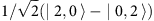—the simplest example of the elusive ‘NOON’ state4. Whereas the HOM effect with (massless) photons has been extensively studied in quantum optics (see refs 5, 6 and references therein), two-particle quantum interference with massive particles remains largely unexplored. A matter-wave demonstration of the HOM effect would be a major advance in experimental quantum physics, enabling an expansion of foundational tests of quantum mechanics into previously unexplored regimes.

Here we propose an experiment that can realize the HOM effect with matter waves using a collision of two atomic Bose–Einstein condensates (BECs) (as in refs 7, 8, 9, 10, 11) and a pair of laser-induced Bragg pulses. The HOM interferometer uses pair-correlated atoms from the scattering halo that is generated during the collision through the process of spontaneous four-wave mixing. The pair-correlated atoms are mixed using two separate Bragg pulses12,13 that realize an atom-optics mirror and beam-splitter elements—in analogy with the use of twin-photons from parametric down-conversion in the optical HOM interferometer scheme. The HOM effect is quantified via the measurement of a set of atom–atom pair correlation functions between the output ports of the interferometer. Using stochastic quantum simulations of the collisional dynamics and the application of Bragg pulses, we predict a HOM dip visibility of ~69% for realistic experimental parameters. A visibility larger than 50% is indicative of stronger than classical correlations between the atoms in the scattering halo7,11,14,15,16, which in turn renders our system as a suitable platform for demonstrating a Bell's inequality violation with matter waves using a closely related Rarity–Tapster scheme17.

## Results

### Setup

The schematic diagram of the proposed experiment is shown in Fig. 1. A highly elongated (along the x axis) BEC is initially split into two equal and counterpropagating halves travelling with momenta ±k0 along z in the centre-of-mass frame. Constituent atoms undergo binary elastic collisions that produce a nearly spherical s-wave scattering halo of radius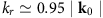(ref. 8) in momentum space due to energy and momentum conservation. The elongated condensates have a disk-shaped density distribution in momentum space, shown in Fig. 1b on the north and south poles of the halo. After the end of the collision (which in this geometry corresponds to complete spatial separation of the condensates in position space), we apply two counterpropagating lasers along the x axis whose intensity and frequency are tuned to act as a resonant Bragg π-pulse with respect to two diametrically opposing momentum modes, k1 and k2=−k1, situated on the equatorial plane of the halo and satisfying |k1,2|=kr.

Previous experiments and theoretical work7,9,10,11,18,19,20,21,22,23 have shown the existence of strong atom–atom correlation between such diametrically opposite modes, similar to the correlation between twin-photons in parametric down-conversion. Applying the Bragg π-pulse to the collisional halo replicates an optical mirror and reverses the trajectories of the scattered atoms with momenta k1 and k2, and a finite region around them. We assume that the pulse is tuned to operate in the so-called Bragg regime of the Kapitza-Dirac effect13,24 (diffraction of a matter wave from a standing light field), corresponding to conditions in which second- and higher-order diffractions are suppressed. The system is then allowed to propagate freely for a duration so that the targeted atomic wave-packets regain spatial overlap in position space. We then apply a second Bragg pulse—a π/2-pulse—to replicate an optical 50:50 beam splitter, which is again targeted to couple k1 and k2, thus realising the HOM interferometer.

The timeline of the proposed experiment is illustrated in Fig. 2a, whereas the results of numerical simulations (see Methods) of the collision dynamics and the application of Bragg pulses are shown in Fig. 2b–d: panel b shows the equatorial slice of the momentum-space density distribution n(k,t) of the scattering halo at the end of collision; panels c and d show the halo density after the application of the π and π/2 pulses, respectively. The ‘banana’ shaped regions in panel c correspond to ‘kicked’ populations between the targeted momenta around k1 and k2 in the original scattering halo, whereas panel d shows the density distribution after mixing. The density modulation in panel c is simply the result of interference between the residual and transferred atomic populations after the π-pulse upon their recombination on the beam splitter. The residual population is due to the fact that the pairs of off-resonance modes in these parts of the halo (which are coupled by the same Bragg pulses as they share the same momentum difference 2kr as the resonant modes k1 and k2) no longer satisfy the perfect Bragg-resonance condition and therefore the population transfer during the π-pulse is not 100% efficient. As these components have unequal absolute momenta, their amplitudes accumulate a nonzero relative phase due to phase dispersion during the free propagation. The accrued relative phase results in interference fringes upon the recombination on the beam splitter, with an approximate period of.Figure 2: Timeline of the proposed experiment and snapshots of the collisional halo from the numerical simulations.

Owing to the indistinguishability of the paths of the Bragg-resonant modes k1 and k2 through the beam splitter and the resulting destructive quantum interference, a measurement of coincidence counts between the atomic populations in these modes will reveal a suppression compared with the background level. To reveal the full structure of the HOM dip, including the background level where no quantum interference occurs, we must introduce path distinguishability between the k1 and k2 modes. One way to achieve this, which would be in a direct analogy with shifting the beam splitter in the optical HOM scheme, is to change the Bragg-pulse resonance condition from the (k1, k2) pair to (k1, k2+êxδk), where êx is the unit vector in the x-direction. The approach to the background coincidence rate between the populations in the k1 and k2 modes would then correspond to performing the same experiment for increasingly large displacements δk. Taking into account that acquiring statistically significant results for each δk requires repeated runs of the experiment (typically thousands), this measurement protocol could potentially pose a significant practical challenge because of the very large total number of experimental runs required.

### Proposed measurement protocol

To overcome this challenge, we propose an alternative measurement protocol that can reveal the full structure of the HOM dip from just one Bragg-resonance condition, requiring only one set of experimental runs. The protocol takes advantage of the broadband, multimode nature of the scattering halo and the fact that the original Bragg-pulse couples not only the targeted momentum modes k1 and k2, but also many other pairs of modes that follow distinguishable paths through the beam splitter. One such pair, k3=(kx,ky,kz)=kr(cos(θ),sin(θ),0) and k4=−k3, located on the halo peak, is shown in Fig. 3a and corresponds to a rotation by angle θ away from k1 and k2. The modes k3 and k4 are equivalent to the original pair in the sense of their quantum statistical properties and therefore, these modes can be used for the measurement of the background level of coincidence counts, instead of physically altering the paths of the k1 and k2 modes. The angle θ now serves the role of the ‘displacement’ parameter that scans through the shape of the HOM dip. A topologically equivalent optical scheme is shown in Fig. 3b,c, which is in turn similar to the one analysed in the study by Rarity and Tapster25 using a broadband source of angle-separated pair-photons and directionally asymmetric apertures.Figure 3: Schematic of matter-wave momentum modes affected by the Bragg pulses and the topologically equivalent optical setups.

In the proposed protocol, detection (after the final Bragg pulse) of atom coincidences at the pair of originally correlated momenta k3 and k4 corresponds to both paths being separately reflected on the beam splitter (Fig. 3c). Apart from this outcome, we need to take into account the coincidences between the respective Bragg-partner momenta, k6 and k5 (separated, respectively, from k3 and k4 by the same difference 2kr as k1 from k2). Coincidences at k6 and k5 correspond to atoms of the originally correlated momenta k3 and k4 being both transmitted through the beam splitter (Fig. 3c). Finally, in order to take into account all possible channels contributing to coincidence counts between the two arms of the interferometer, we need to measure coincidences between k3 and k6, as well as between k4 and k5. This ensures that the total detected flux at the output ports of the beam splitter matches the total input flux. In addition to this, we normalize the bare coincidence counts to the product of single-detector count rates, that is, the product of the average number of atoms in the two output arms of the interferometer. We use the normalized correlation function as the total population in the four relevant modes varies as the angle θ is increased, implying that the raw coincidence rates are not a suitable quantity to compare at different angles.

### HOM effect and visibility of the dip

With this measurement protocol in mind, we quantify the HOM effect using the normalized second-order correlation function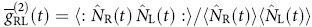after the π/2-pulse concludes at t=t4. Here,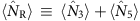andcorrespond to the number of atoms detected, respectively, on the two (right and left) output ports of the beam splitter, with the detection bins centred around the four momenta of interest ki (i=3, 4, 5 and 6), for any given angle θ (Fig. 2e). More specifically,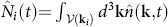is the atom number operator in the integration volume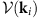centred around ki, whereis the momentum-space density operator, with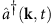and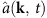the corresponding creation and annihilation operators (the Fourier components of the field operators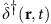and, see Methods). The double-colon notation in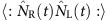indicates normal ordering of the creation and annihilation operators.

The integrated form of the second-order correlation function, which quantifies the correlations in terms of atom number coincidences in detection bins of certain size rather than in terms of local density-density correlations, accounts for limitations in the experimental detector resolution, in addition to improving the signal-to-noise ratio that is typically low owing to the relatively low density of the scattering halo; in typical condensate collision experiments and in our simulations, the low density translates to a typical halo-mode occupation of ~0.1. We choose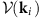to be a rectangular box with dimensions corresponding to the r.m.s. width of the initial momentum distribution of the trapped condensate, which is a reasonable approximation to the mode (or coherence) volume in the scattering halo10,22.

The second-order correlation function, quantifying the HOM effect as a function of the path-distinguishability angle θ, is shown in Fig. 4. For θ=0, where k3(4)=k1(2), we observe maximum suppression of coincidence counts relative to the background level because of the indistinguishability of the paths. As we increase |θ|>0, we no longer mix k3 and k4 as a pair and their paths through the beam splitter become distinguishable; the path interference is lost, and we observe an increase in the magnitude of the correlation function to the background level. We quantify the visibility of the HOM dip via, whereoccurs for θ=0 and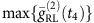for sufficiently large θ such that momenta k5,6 lie outside the scattering halo. Owing to the oscillatory nature of the wings (see below) we taketo correspond to the mean offor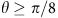. Using this definition, we measure a visibility of, where the uncertainty of ±0.08 corresponds to taking into account the full fluctuations of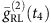about the mean in the wings rather than fitting the oscillations (Supplementary Fig. 1 and Supplementary Notes 1 and 2). The visibility >0.5 is consistent with the nonclassical effect of violation of Cauchy–Schwarz inequality with matter waves22, observed recently in condensate collision experiments11. The exact relationship between the visibility and the Cauchy–Schwarz inequality is discussed further in Supplementary Note 3, as are simple (approximate) analytic estimates of the magnitude of the HOM dip visibility (Supplementary Notes 1–5 and Supplementary Fig. 2).

### Width of the HOM dip

The broadband, multimode nature of the scattering halo implies that the range of the path-length difference over which the HOM effect can be observed is determined by the spectral width of the density profile of the scattering halo. Therefore, the width of the HOM dip is related to the width of the halo density. This is similar to the situation analysed in Rarity and Tapster25 using pair-photons from a broadband parametric down-converter. The angular width of the HOM dip extracted from Fig. 4 is approximately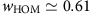radians, which is indeed close to the width (full width at half maximum) of the scattering halo in the relevant direction,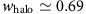radians (see also Supplementary Note 2 for simple analytic estimates). The same multimode nature of the scattering halo contributes to the oscillatory behaviour in the wings of the HOM dip profile: here we mix halo modes with unequal absolute momenta and the resulting phase dispersion from free propagation leads to oscillations similar to those observed with two-colour photons25.

### Comparison with optical parametric down-conversion

We emphasize that the input state in our matter-wave HOM interferometer is subtly different from the idealized twin-Fock state |1,1› used in the simplest analytic descriptions of the optical HOM effect. This idealized state stems from treating the process of spontaneous optical parametric down-conversion (SPDC) in the weak-gain regime. We illustrate this approximation by considering a two-mode toy model of the process, which in the undepleted pump approximation is described by the Hamiltonian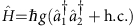that produces perfectly correlated photons in the â1 and â2 modes, where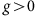is a gain coefficient related to the quadratic nonlinearity of the medium and the amplitude of the coherent pump beam. (In the context of condensate collisions, the coupling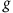corresponds to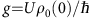at the same level of ‘undepleted pump’ approximation10,22; see Methods for the definitions of U and ρ0.) The full output state of the SPDC process in the Schrödinger picture is given by, where α=tanh(gt) and t is the interaction time26. In the weak-gain regime, corresponding to, this state is well approximated by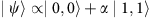, that is, by truncating the expansion of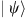and neglecting the contribution of the |2,2› and higher n components. This regime corresponds to mode populations being much >1,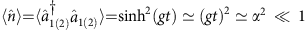. The truncated state itself is qualitatively identical to the idealized state |1,1› as an input to the HOM interferometer: both result in a HOM dip minimum of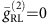andin the wings (wherefor the |1,1› case), with the resulting maximum visibility of V=1. If, on the other hand, the contribution of the |2,2› and higher n components is not negligible (which is the case, for example, of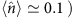then the raw coincidence counts at the HOM dip and the respective normalized correlation function no longer equal to zero; in fact, the full SPDC state for arbitrary α<1 leads to a HOM dip minimum ofand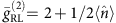in the wings, which in turn results in a reduced visibility of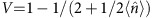. Despite this reduction, the visibility is still very close to V=1 in the weak-gain regime (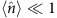), where it scales as.

The process of four-wave mixing of matter waves gives rise to an output state analogous to the above SPDC state for each pair of correlated modes (see, for example, refs 10, 22 and Supplementary Note 1). Indeed, the fraction of atoms converted from the source BEC to all scattering modes is typically <5%, which justifies the use of the undepleted pump approximation. The typical occupation numbers of the scattered modes are, however, beyond the extreme of a very weak gain. In our simulations, the mode occupation on the scattering halo is on the order of 0.1 and therefore, even in the simplified analytical treatment of the process, the output state of any given pair of correlated modes cannot be approximated by the truncated state |0,0›+α|1,1› or indeed the idealized twin-Fock state |1,1›.

### Scaling with mode population and experimental considerations

At the basic level, our proposal only relies on the existence of the aforementioned pair-correlations between scattered atoms, with the strength of the correlations affecting the visibility of the HOM dip. For a sufficiently homogeneous source BEC22,27, the correlations and thus the visibility V effectively depend only on the average mode population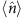in the scattering halo, with a scaling of V ongiven byby our analytic model. Dependence ofon system parameters such as the total number of atoms in the initial BEC, trap frequencies and collision duration is well understood both theoretically and experimentally7,8,10,11, and each can be sufficiently controlled such that a suitable mode population of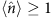can, in principle, be targeted. There lies, however, a need for optimization: very small populations are preferred for higher visibility, but they inevitably lead to a low signal to noise, hence requiring a potentially very large number of experimental runs for acquiring statistically significant data. Large occupations, on the other hand, lead to higher signal to noise, but also to a degradation of the visibility towards the nonclassical threshold of V=0.5. The mode population of ~0.1 resulting from our numerical simulations appears to be a reasonable compromise; following the scaling of the visibility withpredicted by the simple analytic model, it appears that one could safely increase the population to ~0.2 before a nonclassical threshold is reached to within a typical uncertainty of ~12% (as per quoted value of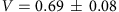) obtained through our simulations.

The proposal is also robust to other experimental considerations such as the implementation of the Bragg pulses; for example, one may use square Bragg pulses rather than Gaussians. Furthermore, experimental control of the Bragg pulses is sufficiently accurate to avoid any degradation of the dip visibility. Modifying the relative timing of the π and π/2 pulses by few percent in our simulations does not explicitly affect the dip visibility, rather only the period of the oscillations in the wings of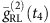. This may lead to a systematic change in the calculated dip visibility, however, this is overwhelmed by the uncertainty of 12% that accounts for the fluctuations ofabout the mean.

Importantly, we expect that the fundamentally new aspects of the matter-wave setup, namely the multimode nature of the scattering halo and the differences from the archetypical HOM input state of |1,1›, as well as the specific measurement protocol we have proposed for dealing with these new aspects, are broadly applicable to other related matter-wave setups that generate pair-correlated atoms. These include molecular dissociation19, an elongated BEC in a parametrically shaken trap14, or degenerate four-wave mixing in an optical lattice28,29. In the present work, we focus on condensate collisions only because of the accurate characterization, both experimental and theoretical, of the atom–atom correlations, including in a variety of collision geometries7,8,9,10,11.

## Discussion

In summary, we have shown that an atom-optics analogue of the HOM effect can be realised using colliding condensates and laser-induced Bragg pulses. The HOM dip visibility >50% implies that the atom–atom correlations in this process cannot be described by classical stochastic random variables. Generation and detection of such quantum correlations in matter waves can serve as precursors to stronger tests of quantum mechanics such as those implied by a Bell inequality violation and the Einstein–Podolsky–Rosen paradox30. In particular, the experimental demonstration of the atom-optics HOM effect would serve as a suitable starting point to experimentally demonstrate a violation of a Bell inequality using an atom-optics adaptation of the Rarity–Tapster setup17. In this setup, one would tune the Bragg pulses as to realize two separate HOM interferometer arms, enabling to mix two angle-resolved pairs of momentum modes from the collisional halo, such as (k,q) and (−k,−q), which would then form the basis of a Bell state(ref. 31).

## Methods

### Stochastic Bogoliubov approach for simulations

To simulate the collision dynamics, we use the time-dependent stochastic Bogoliubov approach8,23 used previously to accurately model a number of condensate collision experiments8,7,11. In this approach, the atomic field operator is split into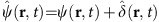, where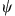is the mean-field component describing the source condensates and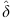is the fluctuating component (treated to lowest order in perturbation theory) describing the scattered atoms. The mean-field component evolves according to the standard time-dependent Gross–Pitaevskii equation, where the initial state is taken in the form of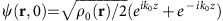. This models an instantaneous splitting at t=0 of a zero-temperature condensate in a coherent state into two halves that subsequently evolve in free space, where ρ0(r) is the particle number density of the initial (trapped) sample before splitting.

The fluctuating component is simulated using the stochastic counterpart of the Heisenberg operator equations of motion8,22,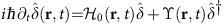, in the positive P-representation with the vacuum initial state. Here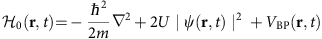represents the kinetic energy term, an effective mean-field potential, plus the lattice potential VBP(r,t) imposed by the Bragg pulses, whereas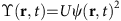is an effective coupling responsible for the spontaneous pair-production of scattered atoms. The interaction constant U is given by U=4π2a/m, where m is the atomic mass and a is the s-wave scattering length.

### Details of Bragg pulses

The Bragg pulses are realised by two interfering laser beams (assumed for simplicity to have a uniform intensity across the atomic cloud and zero relative phase) that create a periodic lattice potential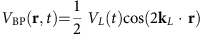, where VL(t) is the lattice depth and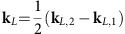is the lattice vector determined by the wave vectors kL,i (i=1, 2) of the two lasers, and tuned to |kL|=kr. The Bragg pulses couple momentum modes ki and kj=ki−2kL, satisfying momentum and energy conservation (up to a finite width owing to energy-time uncertainty24). The lattice depth is ramped up (down) according to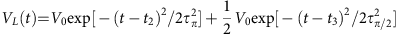, where t2(3) is the pulse centre, whereas τπ(π/2) is the pulse duration that governs the transfer of atomic population between the targeted momentum modes: a π-pulse is defined by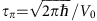and converts the entire population from one momentum mode to the other, whereas a π/2-pulse is defined by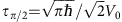and converts only half of the population.

### Aspects of measurement after expansion

In practice, the atom–atom correlations quantifying the HOM interference are measured in position space after the low-density scattering halo expands ballistically in free space and falls under gravity onto an atom detector. The detector records the arrival times and positions of individual atoms, which is literally the case for metastable helium atoms considered here7,8,9,11,32. The arrival times and positions are used to reconstruct the three-dimensional velocity (momentum) distribution before expansion, as well as the atom–atom coincidences for any desired pair of momentum vectors. In our simulations and the proposed geometry of the experiment, the entire system (including the Bragg pulses) maintains reflectional symmetry about the yz-plane, with z being the vertical direction. Therefore, the effect of gravity can be completely ignored as it does not introduce any asymmetry to the momentum distribution of the atoms and their correlations on the equatorial plane of the halo or indeed any other plane parallel to it.